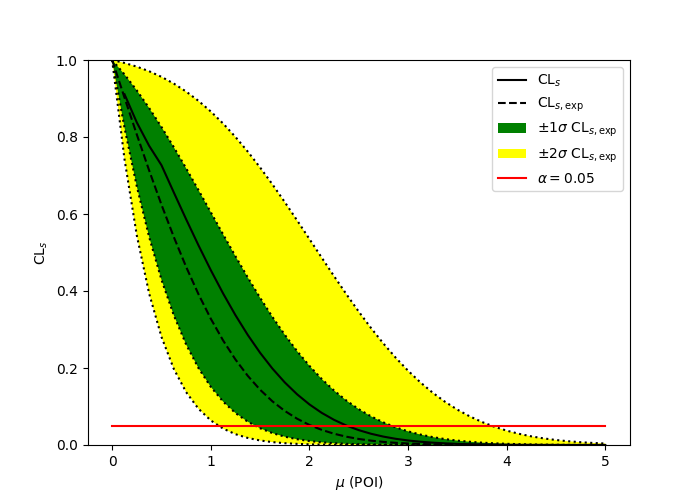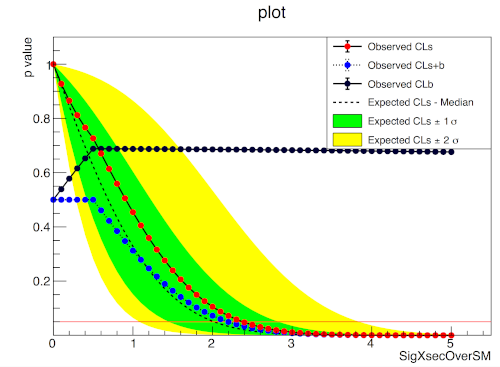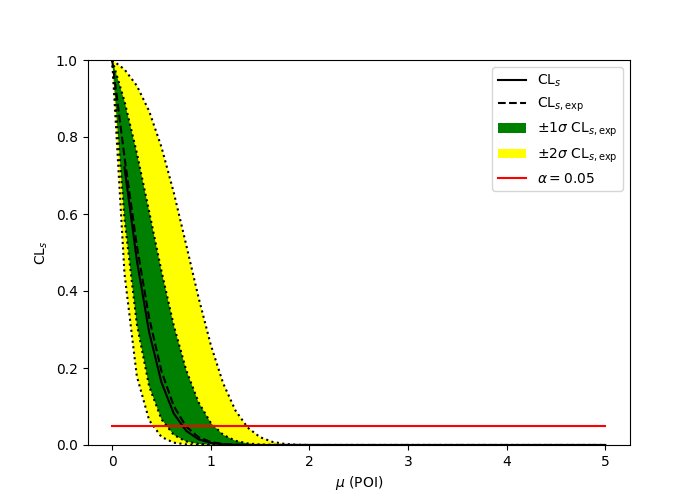Fork me on GitHub# pure-python fitting/limit-setting/interval estimation HistFactory-style¶

The HistFactory p.d.f. template [CERN-OPEN-2012-016] is per-se independent of its implementation in ROOT and sometimes, it’s useful to be able to run statistical analysis outside of ROOT, RooFit, RooStats framework.

This repo is a pure-python implementation of that statistical model for multi-bin histogram-based analysis and its interval estimation is based on the asymptotic formulas of “Asymptotic formulae for likelihood-based tests of new physics” [arXiv:1007.1727]. The aim is also to support modern computational graph libraries such as PyTorch and TensorFlow in order to make use of features such as autodifferentiation and GPU acceleration.

## Hello World¶

>>> import pyhf
>>> model = pyhf.simplemodels.hepdata_like(signal_data=[12.0, 11.0], bkg_data=[50.0, 52.0], bkg_uncerts=[3.0, 7.0])
>>> data = [51, 48] + model.config.auxdata
>>> test_mu = 1.0
>>> CLs_obs, CLs_exp = pyhf.infer.hypotest(test_mu, data, model, qtilde=True, return_expected=True)
>>> print(f"Observed: {CLs_obs}, Expected: {CLs_exp}")
Observed: 0.05251497423736956, Expected: 0.06445320535890459


## What does it support¶

Implemented variations:
• ☑ HistoSys

• ☑ OverallSys

• ☑ ShapeSys

• ☑ NormFactor

• ☑ Multiple Channels

• ☑ Import from XML + ROOT via uproot

• ☑ ShapeFactor

• ☑ StatError

• ☑ Lumi Uncertainty

Computational Backends:
• ☑ NumPy

• ☑ PyTorch

• ☑ TensorFlow

• ☑ JAX

Optimizers:
• ☑ SciPy (scipy.optimize)

• ☑ MINUIT (iminuit)

All backends can be used in combination with all optimizers. Custom user backends and optimizers can be used as well.

## Todo¶

• ☐ StatConfig

• ☐ Non-asymptotic calculators

results obtained from this package are validated against output computed from HistFactory workspaces

## A one bin example¶

import pyhf
import numpy as np
import matplotlib.pyplot as plt
import pyhf.contrib.viz.brazil

pyhf.set_backend("numpy")
model = pyhf.simplemodels.hepdata_like(
signal_data=[10.0], bkg_data=[50.0], bkg_uncerts=[7.0]
)
data = [55.0] + model.config.auxdata

poi_vals = np.linspace(0, 5, 41)
results = [
pyhf.infer.hypotest(test_poi, data, model, qtilde=True, return_expected_set=True)
for test_poi in poi_vals
]

fig, ax = plt.subplots()
fig.set_size_inches(7, 5)
ax.set_xlabel(r"$\mu$ (POI)")
ax.set_ylabel(r"$\mathrm{CL}_{s}$")
pyhf.contrib.viz.brazil.plot_results(ax, poi_vals, results)


pyhfROOT## A two bin example¶

import pyhf
import numpy as np
import matplotlib.pyplot as plt
import pyhf.contrib.viz.brazil

pyhf.set_backend("numpy")
model = pyhf.simplemodels.hepdata_like(
signal_data=[30.0, 45.0], bkg_data=[100.0, 150.0], bkg_uncerts=[15.0, 20.0]
)
data = [100.0, 145.0] + model.config.auxdata

poi_vals = np.linspace(0, 5, 41)
results = [
pyhf.infer.hypotest(test_poi, data, model, qtilde=True, return_expected_set=True)
for test_poi in poi_vals
]

fig, ax = plt.subplots()
fig.set_size_inches(7, 5)
ax.set_xlabel(r"$\mu$ (POI)")
ax.set_ylabel(r"$\mathrm{CL}_{s}$")
pyhf.contrib.viz.brazil.plot_results(ax, poi_vals, results)


pyhfROOT## Installation¶

To install pyhf from PyPI with the NumPy backend run

python -m pip install pyhf


and to install pyhf with all additional backends run

python -m pip install pyhf[backends]


or a subset of the options.

To uninstall run

python -m pip uninstall pyhf


## Questions¶

If you have a question about the use of pyhf not covered in the documentation, please ask a question on Stack Overflow with the [pyhf] tag, which the pyhf dev team watches.If you believe you have found a bug in pyhf, please report it in the GitHub Issues.

## Citation¶

As noted in Use and Citations, the preferred BibTeX entry for citation of pyhf is

@software{pyhf,
author = "{Heinrich, Lukas and Feickert, Matthew and Stark, Giordon}",
title = "{pyhf: v0.5.2}",
version = {0.5.2},
doi = {10.5281/zenodo.1169739},
url = {https://github.com/scikit-hep/pyhf},
}


## Authors¶

pyhf is openly developed by Lukas Heinrich, Matthew Feickert, and Giordon Stark.

Please check the contribution statistics for a list of contributors.

## Milestones¶

• 2020-07-28: 1000 GitHub issues and pull requests. (See PR #1000)

## Acknowledgements¶

Matthew Feickert has received support to work on pyhf provided by NSF cooperative agreement OAC-1836650 (IRIS-HEP) and grant OAC-1450377 (DIANA/HEP).